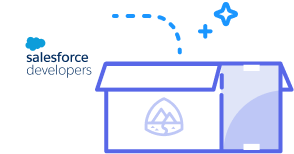function readOnly(count){ }Starting November 20, the site will be set to read-only. On December 4, 2023,
forum discussions will move to the Trailblazer Community.
ShowAll Questionssorted byDate PostedSree Salesforce

# whats is call by value and call by referenceBest Answer chosen by Sree SalesforceAshish_SFDC
Hi Sri,

call by value = only the value of the variable is given as a parameter. When you change the value in the function, nothing happens to the variable in the structure that calls our function.
All primitive data types are passed by value.(eg string, boolean, date, datetime, decimal etc)

call by reference = the reference to the variable is given as a parameter. This is the normal case. Anything the function changes with it, goes right back to the variable in the structure that calls our function.
Non primitive data types are passed by refernce(eg list, set, map)

Regards,
AshishAshish_SFDC
Hi Sri,

call by value = only the value of the variable is given as a parameter. When you change the value in the function, nothing happens to the variable in the structure that calls our function.
All primitive data types are passed by value.(eg string, boolean, date, datetime, decimal etc)

call by reference = the reference to the variable is given as a parameter. This is the normal case. Anything the function changes with it, goes right back to the variable in the structure that calls our function.
Non primitive data types are passed by refernce(eg list, set, map)

Regards,
Ashish
This was selected as the best answerForce Techiek3
Hi Sri

Call by value: In the call by value method, the called function creates a new set of variables in stack and copies the values of the arguments into them.
Example: Program showing the Call by Value method

void swap(int x, int y)
{
int z;
z = x;
x = y;
y = z;
printf("Swapped values are a = %d and b = %d", x, y);
}

void main()
{
int a = 7, b = 4;
printf("Original values are a = %d and b = %d", a, b);
swap(a, b);
printf("The values after swap are a = %d and b = %d", a, b);
}

Output:
Original Values are a = 7 and b = 4
Swapped values are a = 4 and b = 7
The values after swap are a = 7 and b = 4
This happens because when function swap() is invoked, the values of a and b gets copied onto x and y. The function actually swaps x and y while the values of the original variables a and b remain intact.
Here is the block diagram describing disassembly steps, call stack and argument variables. Calling swap (a, b) can be split into some assembly steps like-

push value of b
push value of a
call functionCall by value - describing disassembly steps, call stack and argument variables

Here one point to note is x and y are in stack. Value of a and b will be copied to x and y. Inside swap() value of x and y will be interchanged but it will not affect a and b in the main().

Call by reference: In the call by reference method, instead of passing values to the function being called, references/pointers to the original variables are passed.
Example: Program showing the Call by Reference method

void swap(int *x, int *y)
{
int z;
z = *x;
*x = *y;
*y = z;
printf("Swapped values are a = %d and b = %d", *x, *y);
}
void main()
{
int a = 7, b = 4;
printf("Original values are a = %d and b = %d", a, b);
swap(&amp;a, &amp;b);
printf("The values after swap are a = %d and b = %d", a, b);
}

Output:
Original Values are a = 7 and b = 4
Swapped values are a = 4 and b = 7
The values after swap are a = 4 and b = 7
This happens because when function swap() is invoked, it creates a reference for the first incoming integer a in x and the second incoming integer b in y. Hence the values of the original variables are swapped.
Here is the block diagram describing disassembly steps, call stack and argument variables. Calling swap (&a, &b) can be split into some assembly steps like-